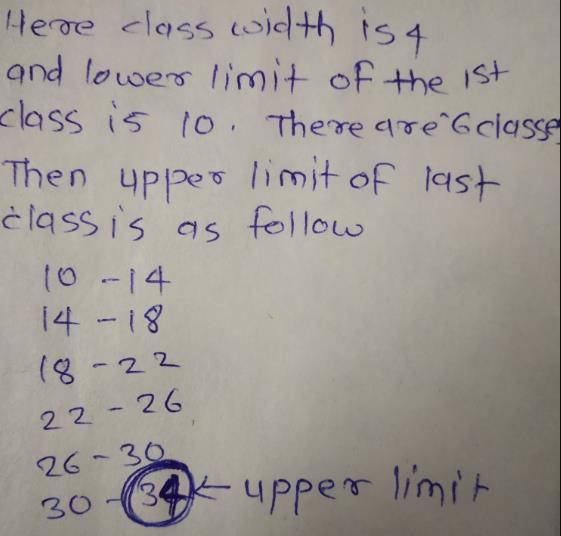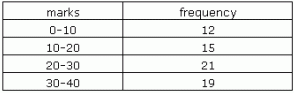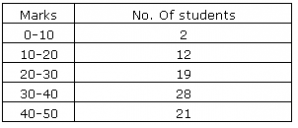# Test: Graphical Representation Of Data

## 5 Questions MCQ Test Mathematics (Maths) Class 9 | Test: Graphical Representation Of Data

Description
This mock test of Test: Graphical Representation Of Data for Class 9 helps you for every Class 9 entrance exam. This contains 5 Multiple Choice Questions for Class 9 Test: Graphical Representation Of Data (mcq) to study with solutions a complete question bank. The solved questions answers in this Test: Graphical Representation Of Data quiz give you a good mix of easy questions and tough questions. Class 9 students definitely take this Test: Graphical Representation Of Data exercise for a better result in the exam. You can find other Test: Graphical Representation Of Data extra questions, long questions & short questions for Class 9 on EduRev as well by searching above.
QUESTION: 1

Solution:
QUESTION: 2

Solution:
QUESTION: 3

### In a frequency distribution, the class-width is 4 and the lower limit of first class is 10. If there are six classes, the upper limit of last class is

Solution:QUESTION: 4

A frequency polygon is drawn for the following data. So the x-coordinate of the point having y coordinate as 21 isSolution:

A frequency polygon is made by joining the mid points of each bar of a histogram along with 2 imaginary class intervals. Hence, for any point on a frequency polygon the y co-ordinate is the frequency and x co - ordinate is the Class Mark of the interval. In this case, the class mark of the interval 20-30 is 25.

QUESTION: 5

Following table gives marks and number of students of a class:Which point on the frequency polygon will have its y coordinate as 21?

Solution: